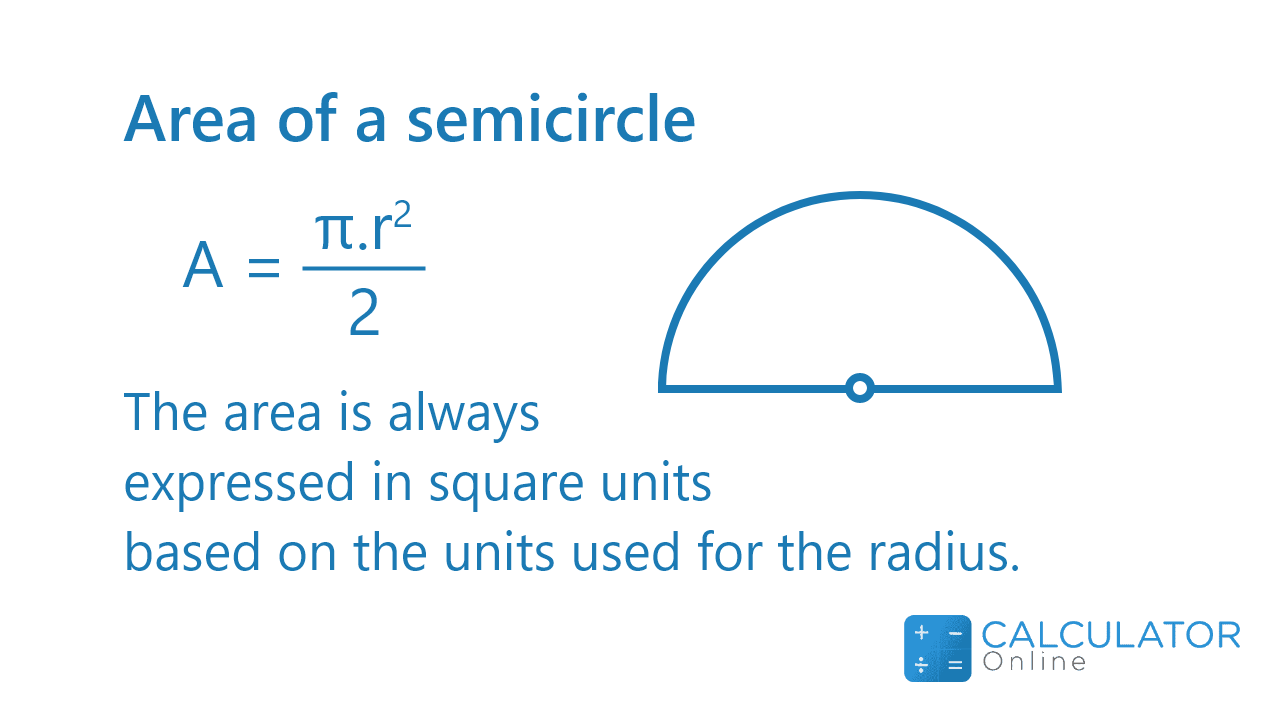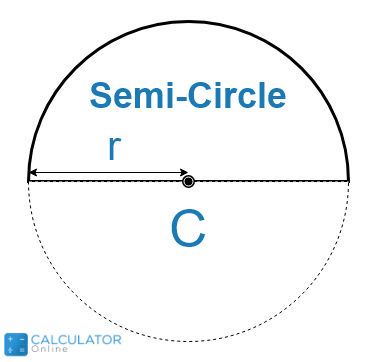•Sign In
• Hire UsUh Oh! It seems you’re using an Ad blocker!

We always struggled to serve you with the best online calculations, thus, there's a humble request to either disable the AD blocker or go with premium plans to use the AD-Free version for calculators.

Or# Area of a Semicircle Calculator

Calculation With:

pi π=

Units

Table of Content

 1 What is priceeight Class? 2 priceeight Class Chart: 3 How to Calculate priceeight Density (Step by Step): 4 Factors that Determine priceeight Classification: 5 What is the purpose of priceeight Class? 6 Are mentioned priceeight Classes verified by the officials? 7 Are priceeight Classes of UPS and FedEx same?

Get the Widget!

Add this calculator to your site and lets users to perform easy calculations.

Feedback

How easy was it to use our calculator? Did you face any problem, tell us!

This area of a semicircle calculator is specifically designed to calculate the area of semicircle. Not only this, but this half circle calculator will also let you determine various parameters associated with this particular geometric figure.

So without wasting time, let’s get down to the article in which we will be covering the semicircle theory in detail.

Stay Focused!

## What Is a Semicircle?

In terms of geometry:

“The semicircle is a shape that is the half of any circle”### How To Construct Semicircle?Right here, what you need to do is to cut the circle into two equal halves. This could be done if you cut it using the centre of the circle. Rest when it comes to the analysis of the semicircle, then it can be done by the aid of this semicircle area calculator.

### Semicircle Formulas:

Here we have general expressions for each and every parameter of the semicircle that are mentioned as follows:

#### Area of a Half Circle:

$$\text{Area_{semicircle}} = \frac{\text{Area of a Circle}}{2}$$

$$\text{Area_{semicircle}} = \frac{\pir^{2}}{2}$$

Whereas if you are interested in finding the area of a full circle, you can also do that with the help of our another circle calculator in no time.
Perimeter of a Semicircle:

$$\text{Perimeter_{semicircle}} = \left(\frac{Circumference}{2}\right) + 2*radius$$

$$\text{Perimeter_{semicircle}} = \frac{2*\pi*r}{2} + 2*r$$

$$\text{Perimeter_{semicircle}} = \pi*r + 2*r$$

Taking r as common:

$$\text{Perimeter_{semicircle}} = r*\left(\pi + 2\right)$$

The same expression is also used by the how to perimeter of a semicircle calculator as well to determine this essential entity.

#### Diameter of a Semicircle:

Usually in a semicircle, the diameter is the base of it. And you can calculate out by using the following expression:

$$Diameter = 2*Radius$$

And when we divide the diameter by the factor of 2, we will get the radius of the semicircle as below:

$$Radius = \frac{Diameter}{2}$$

#### Arc Length of Semicircle:

$$\text{Arc Length of a Semicircle} = \left(2*\pi*r\right) * \frac{180^{\text{o}}}{360^{\text{o}}}$$

Except this, you can determine the arc length for a whole circle body by using our another Arc Length Calculator.

### How To Find The Area of a Semicircle?

This particular part of the read is packed with a few example problems that will let you get a firm grip over the concept. Let’s move towards these now!

Example # 01:

How to find the area of a half circle having a radius of 23?

Solution:

Here we have the area of a semi circle formula as follows:

$$\text{Area_{semicircle}} = \frac{\pi*r^{2}}{2}$$

$$\text{Area_{semicircle}} = \frac{\pi*23^{2}}{2}$$

$$\text{Area_{semicircle}} = \frac{180*529}{2}$$

$$\text{Area_{semicircle}} = \frac{95220}{2}$$

$$\text{Area_{semicircle}} = 47610$$

This free area of a semicircle calculator also goes for finding the same results but saving you a lot of your precious time.

Example # 02:

Determine the perimeter of the half circle with the radius of 2.

Solution:

Here we have:

$$\text{Perimeter_{semicircle}} = r*\left(\pi + 2\right)$$

$$\text{Perimeter_{semicircle}} = 2*\left(\pi + 2\right)$$

$$\text{Perimeter_{semicircle}} = 2*\pi + 2*2$$

$$\text{Perimeter_{semicircle}} = 2*\pi + 4$$

$$\text{Perimeter_{semicircle}} = 2*\left(\pi + 2\right)$$

You can also verify the results with this online perimeter of semicircle calculator in a span of seconds.

### How Area of a Semicircle Calculator Works?

Pop down to know the working operation of this semi circle area calculator through the guide arranged as:

Input:

• First of all, select which parameter you wish to determine from the first drop-down list
• After you make a selection, go for fetching the related asked entities in their designated fields
• After you are done, hit the calculate button

Output:
The free semicircle calculator displays the following results on your device’ screen:

• Diameter of the semicircle
• Area of the semicircle
• Arc Length of the semicircle
• Perimeter of the semicircle

## FAQ’s:

### What is the semicircle angle?

If we talk about the angle made by the triangle inside the semicircle, then this angle would be 90 degrees. This is a right angle and could be determined by using our triangle calculator as well.

### How many semi circles are there in a circle?

A semicircle is a half circle, so there are two semicircles in a complete circle.

### What is a quarter circle called?

You can term the quarter circle as a quadrant.

### What is the longest chord in a semicircle?

The longest chord in a circle is its diameter. You must remember that all chords can never be termed diameter. But on the other hand, all diameters can be considered as chords.

### How is a circle usually named?

Usually, a circle is named by its centre point. The other parameters that are used to define it include diameter, radius, chord, and arc length.

### What is the shortest part of a circle?

The shortest part of any circle or semicircle is made when it is cut by a line segment anywhere.

### What is the use of the semicircle?

Semicircle helps to calculate the geometric and arithmetic means of two lines. That is why this area of a semicircle calculator is designed to determine the parameters that help in finding these means straightaway.

### What are some real life examples of a semicircle?

Some of the most important and widely used semicircle’s applications include:

• Protractor
• Japanese fans
• Train tunnel
• Napkin holder
• Door mat design
• Windows cut in semi circle design

## Conclusion:

The semicircle is a geometrical figure that allows us to understand various architectural map designs. Architects and Engineers make vast use of the concept to nullify any errors involved in the calculations. This is why this best area of a semicircle calculator allows you to

## References:

From the source of wikipedia: Semicircle, Uses, Equation, Arbelos

From the source of khan academy: Area of parts of circles, Area of a circle, Circumference, Partial circle area

From the source of lumen learning: Finding the Area of Irregular Figures, Problems With Circles